# Merge Two pandas DataFrames in Python (6 Examples)

On this page you’ll learn how to join pandas DataFrames in the Python programming language.

Let’s start right away!

## Different Types of Joins Explained

Before we can start with the Python programming examples, we first need to be aware that there are different ways for the merging of DataFrames available.

Four of the most common types of joins are illustrated in the figure below:As you can see, the figure shows two separate data sets at the top and four different combined versions of these data sets at the bottom:

• Inner join: Keep only IDs that are contained in both data sets.
• Outer join: Keep all IDs.
• Left join: Keep only IDs that are contained in the first data set.
• Right join: Keep only IDs that are contained in the second data set.

We will apply all of these different types of joins in the following examples of this tutorial.

Let’s prepare the examples in Python!

## Exemplifying Data & Add-On Libraries

In this tutorial, we’ll use the functions of the pandas library to merge our DataFrames. If we want to use the functions of the pandas library, we first need to load pandas to Python:

`import pandas as pd                                  # Import pandas`

Next, we have to create two separate pandas DataFrames for the examples:

```data1 = pd.DataFrame({"ID":range(101, 106),          # Create first pandas DataFrame
"x1":range(1, 6),
"x2":["a", "b", "c", "d", "e"],
"x3":range(16, 11, - 1)})
print(data1)                                         # Print first pandas DataFrame``````data2 = pd.DataFrame({"ID":range(104, 108),          # Create second pandas DataFrame
"y1":["x", "y", "x", "y"],
"y2":range(8, 1, - 2)})
print(data2)                                         # Print second pandas DataFrame```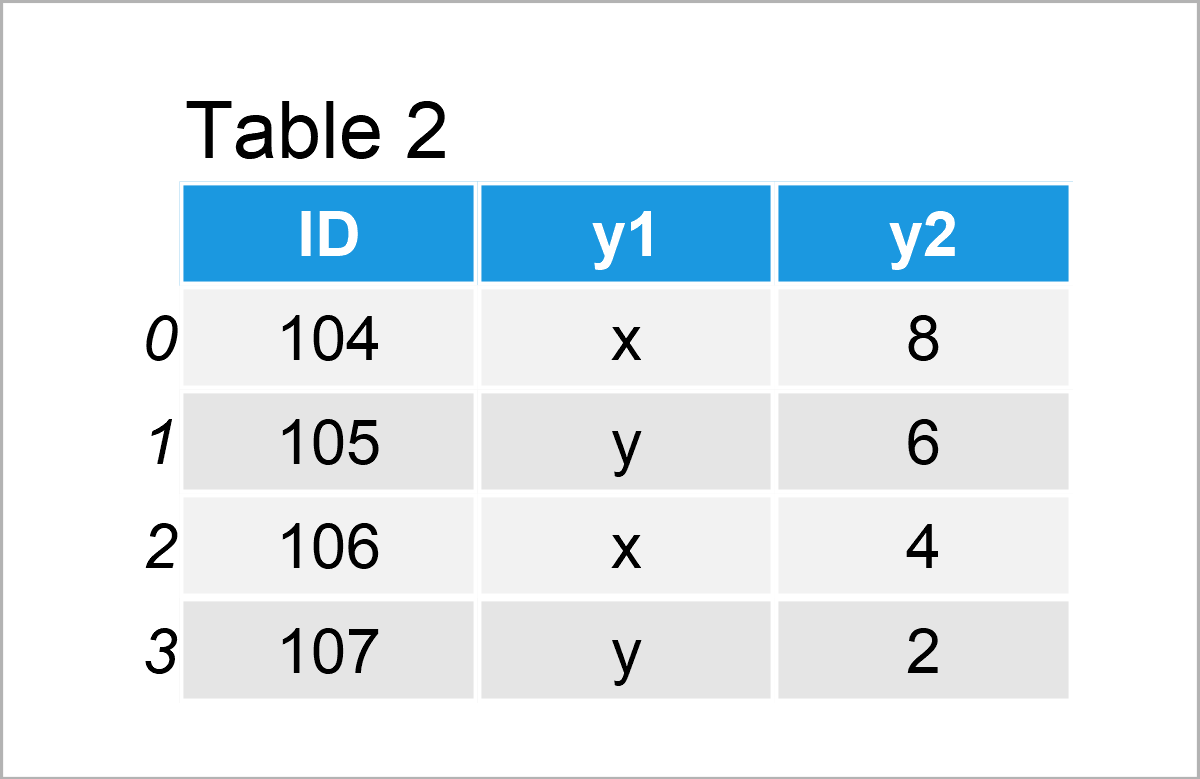The output of the previous Python code is shown in Tables 1 and 2: We have created two pandas DataFrames with different columns and values.

However, both of these DataFrames contain an ID column, and we’ll use this ID column to join our data sets.

Let’s do this!

## Example 1: Merge Two pandas DataFrames Using Inner Join

In Example 1, I’ll demonstrate how to apply an inner join to two pandas DataFrames in Python.

For this, we can use the merge function as shown below. Note that we are specifying the names of our two DataFrames (i.e. data1 and data2) as well as the ID column and the type of join (i.e. “inner”):

```data_inner = pd.merge(data1,                         # Inner join
data2,
on = "ID",
how = "inner")
print(data_inner)                                    # Print merged DataFrame```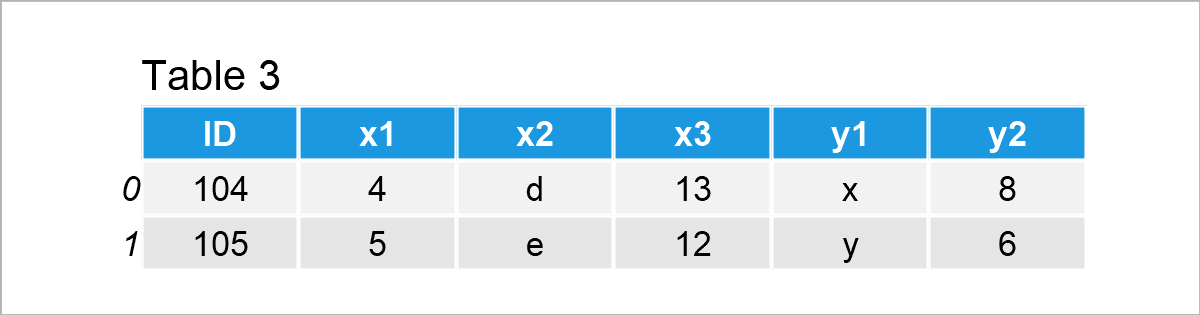After executing the previous code the pandas merged DataFrame revealed in Table 3 has been constructed.

Many rows have been removed from our input DataFrames, since several IDs are only contained in one of the two data sets.

Let’s apply an outer join to keep the most possible data!

## Example 2: Merge Two pandas DataFrames Using Outer Join

The following syntax explains how to use an outer join to union two pandas DataFrames.

All we have to change compared to Example 1 is the how argument (i.e. how = “outer”):

```data_outer = pd.merge(data1,                         # Outer join
data2,
on = "ID",
how = "outer")
print(data_outer)                                    # Print merged DataFrame```As shown in Table 4, we have created another concatenated version of our input DataFrames.

As you can see, all data has been kept. In case an ID existed only in one of the DataFrames, the merge function has assigned an NaN value.

## Example 3: Merge Two pandas DataFrames Using Left Join

In Example 3, I’ll show how to retain only the IDs from the first data set by using a left join.

Again, we only have to adjust the how argument within the merge function:

```data_left = pd.merge(data1,                          # Left join
data2,
on = "ID",
how = "left")
print(data_left)                                     # Print merged DataFrame```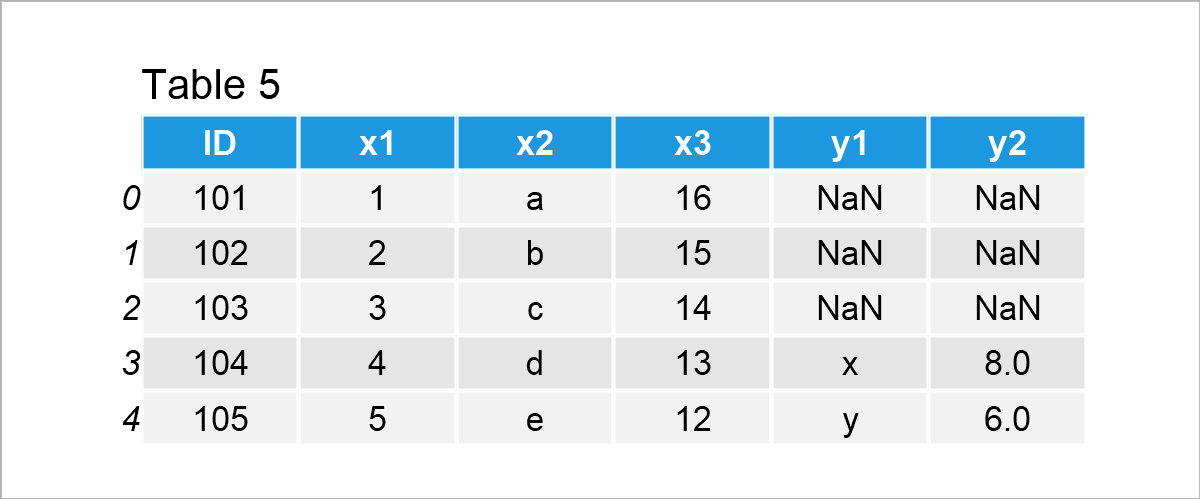As illustrated in Table 5, the previous Python code has managed to add our two input DataFrames together. All data of the first data set has been kept, but the IDs that were only contained in the second data set have been deleted from the final output.

## Example 4: Merge Two pandas DataFrames Using Right Join

We can use a right join to do the opposite as in Example 3, i.e. keeping all IDs of the second DataFrame.

For this, we have to specify the how argument to be equal to “right”:

```data_right = pd.merge(data1,                         # Right join
data2,
on = "ID",
how = "right")
print(data_right)                                    # Print merged DataFrame```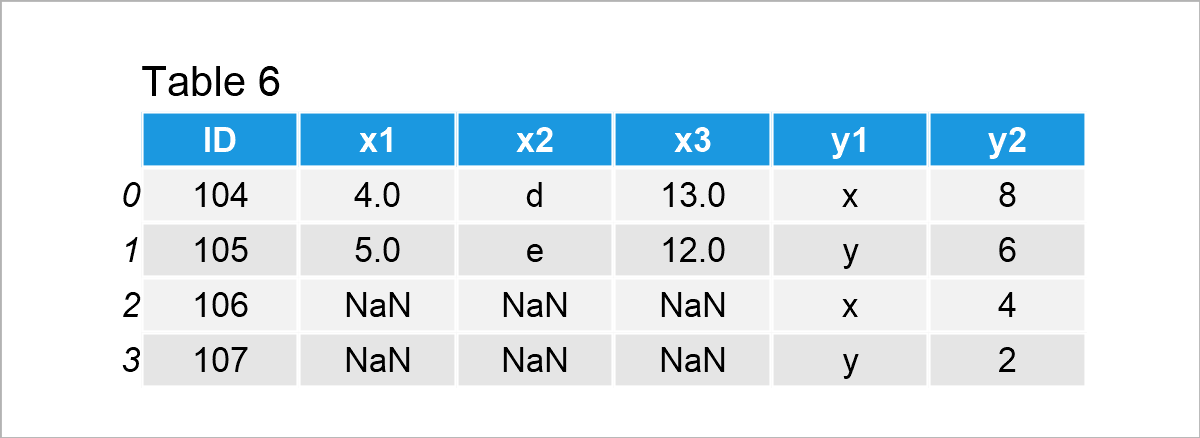As shown in Table 6, the previously shown syntax has created a pandas DataFrame that excludes all IDs that are only contained in the first input data set.

## Example 5: Merge Multiple pandas DataFrames

So far, we have only combined two pandas DataFrames. Example 5 demonstrates how to join more than two DataFrames.

For this, we have to create another pandas DataFrame:

```data3 = pd.DataFrame({"ID":range(102, 110),          # Create third pandas DataFrame
"z1":range(10, 18),
"z2":["z", "b", "z", "z", "d", "z", "d", "a"],
"z3":range(18, 10, - 1)})
print(data3)                                         # Print third pandas DataFrame```In Table 7 you can see that we have created a third input DataFrame that contains an ID column and three other columns called z1, z2, and z3.

Next, we also have to import the reduce function of the functools module:

`from functools import reduce                         # Import reduce function`

In the next step, we can join our three example DataFrames together. Note that we are specifying a list of all our DataFrames within the reduce command:

```data_multi = reduce(lambda left, right:              # Merge three pandas DataFrames
pd.merge(left , right,
on = ["ID"],
how = "outer"),
[data1, data2, data3])
print(data_multi)                                    # Print merged DataFrame```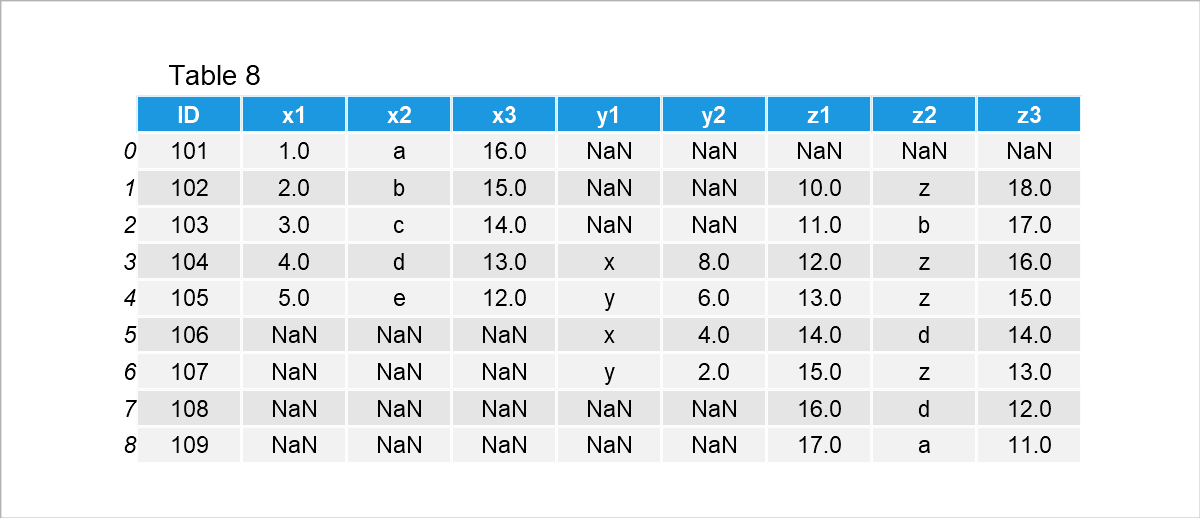The output of the previous Python syntax is shown in Table 8 – We have created a horizontally unified pandas DataFrame containing an outer join of three DataFrames.

## Example 6: Merge pandas DataFrames based on Index

In the previous examples, we have relied on the ID column in our data sets.

In this example, I’ll explain how to use DataFrame indices to concatenate two DataFrames.

Let’s create two further pandas DataFrames in Python:

```data4 = pd.DataFrame({"a1":["yes", "no", "no", "yes", "yes"], # Create fourth pandas DataFrame
"a2":range(15, 20)},
index = list("abcde"))
print(data4)                                         # Print fourth pandas DataFrame``````data5 = pd.DataFrame({"b1":range(10, 5, - 1),        # Create fifth pandas DataFrame
"b2":["b", "bb", "b", "bbb", "b"],
"b3":range(10, 1, - 2)},
index = list("cdefg"))
print(data5)                                         # Print fifth pandas DataFrame```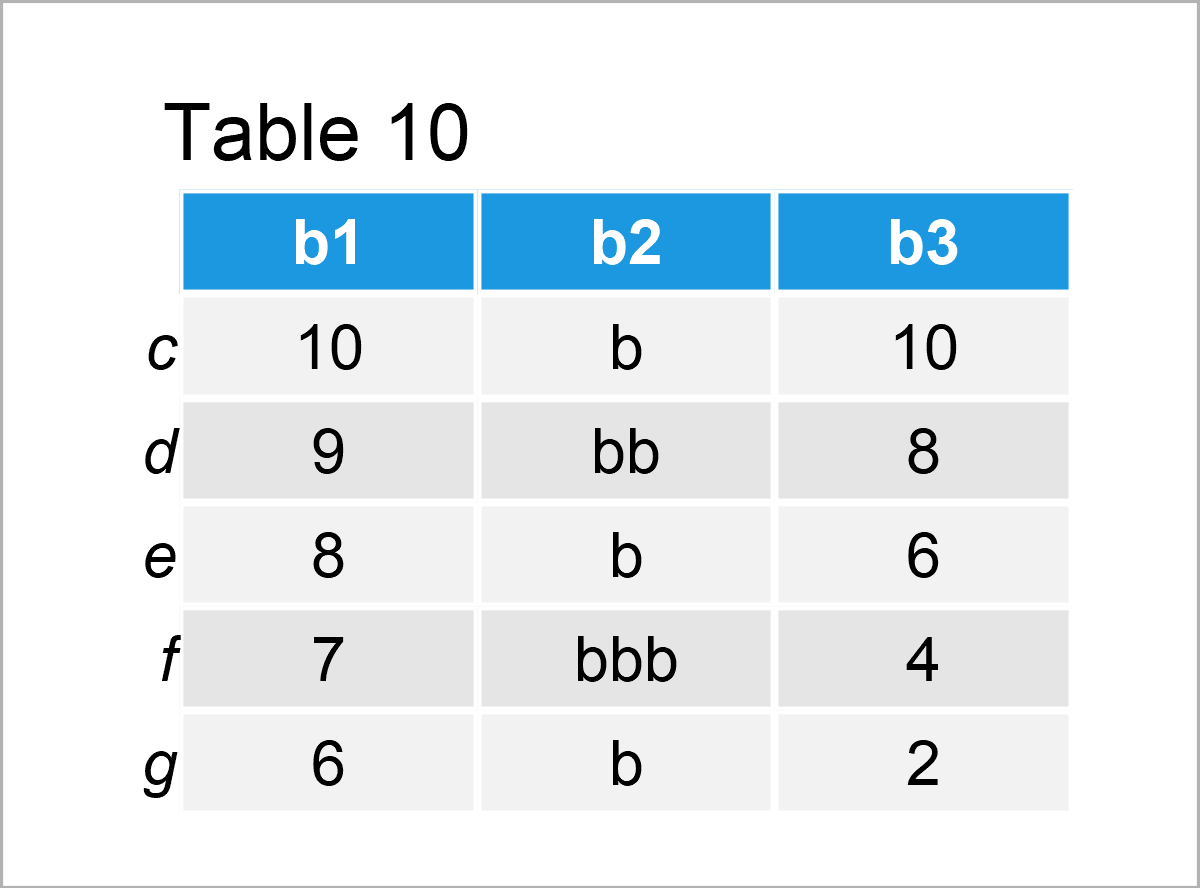After executing the previous Python programming code the two DataFrames shown in Table 10 have been created. Note that both DataFrames contain different row indices.

Next, we can use these index values to merge our DataFrames. For this, we have to specify left_index and right_index arguments to be equal to True. In this specific example, we are using an outer join:

```data_index = pd.merge(data4,                         # Merge DataFrames based on Index
data5,
left_index = True,
right_index = True,
how = "outer")
print(data_index)                                    # Print merged DataFrame```The output of the previous Python syntax is shown in Table 11 – This time we have used the row index names of our two pandas DataFrames to merge our data.

## Video & Further Resources

I have recently published a video on my YouTube channel, which explains the pandas package and the Python programming syntax of this tutorial. You can find the video tutorial below:

Please accept YouTube cookies to play this video. By accepting you will be accessing content from YouTube, a service provided by an external third party.If you accept this notice, your choice will be saved and the page will refresh.

Also, you might have a look at the related articles on statisticsglobe.com. I have published numerous tutorials already: